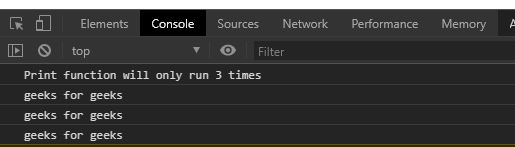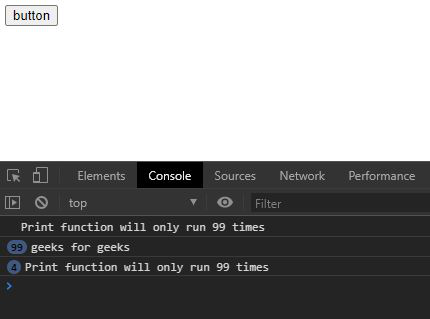# Underscore.js _.before() Function

• Last Updated : 01 Jul, 2022

_.before() function in underscore.js is used to call a particular function a particular number of times. It uses a count variable that keeps the track of number of times the function is called.

Syntax:

`_.before(count, function)`

Parameters:

• Count: It is the number of times a function is to be called.
• Function: It is the function that runs the count times.

Return: This function returns  the count of the function call.

Note: Please Link the underscore CDN before using this code directly in the browser through the code.

Example 1:

## html

 ` ` `<``html``> ` `  ``<``head``> ` `    ``<``script` `src =  ` `"https://cdnjs.cloudflare.com/ajax/libs/underscore.js/1.9.1/underscore-min.js" > ` `    `` ` `  `` ` `  ``<``body``> ` `    ``<``script``>` `      ``let print=()=>{` `        ``console.log("geeks for geeks")` `      ``}` `      ``console.log(`Print function will only run 3 times`)` `      ``//the print function cannot run more than 3 times` `      ``count=_.before(4, print);` `      ``//calling count function more than 3 times` `      ``count();` `      ``count();` `      ``count();` `      ``count();` `      ``count();` `      ``count();` `      ``count();` `    ``` `  `` ` ``

Output:Example 2:

It is clear from the example that if The button is clicked again and again than print function will not be working as it as already reached the count.

## html

 ` ` `<``html``> ` `  ``<``head``> ` `    ``<``script` `src =  ` `"https://cdnjs.cloudflare.com/ajax/libs/underscore.js/1.9.1/underscore-min.js" > ` `    `` ` `   `` ` `  ``<``body``> ` `    ``<``button` `id="btn">button` `    ``<``script``>` `      ``let print=()=>{` `        ``console.log("geeks for geeks")` `      ``}` `      ``//the print function cannot run more than 99 times` `      ``count=_.before(100, print);` `      ``//calling count function more than 99 times` `      ``let btn=document.querySelector("#btn");` `      ``let run=()=>{` `      ``console.log(`Print function will only run 99 times`)` `        ``for(let i=0; i<``5000``; i++){` `          ``count()` `        ``}` `      ``}` `      ``btn.addEventListener("click", run)` `    ``` `  `` ` ``

Output:My Personal Notes arrow_drop_up
Recommended Articles
Page :